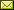## The Cayley-Dickson Construction in ACL2

 John Cowles (University of Wyoming) Ruben Gamboa (The Cayley-Dickson Construction in ACL2)

 The Cayley-Dickson Construction is a generalization of the familiar construction of the complex numbers from pairs of real numbers. The complex numbers can be viewed as two-dimensional vectors equipped with a multiplication. The construction can be used to construct, not only the two-dimensional Complex Numbers, but also the four-dimensional Quaternions and the eight-dimensional Octonions. Each of these vector spaces has a vector multiplication, v_1*v_2, that satisfies: 1. Each nonzero vector has a multiplicative inverse. 2. For the Euclidean length of a vector |v|, |v_1 * v_2| = |v_1| |v2|. Real numbers can also be viewed as (one-dimensional) vectors with the above two properties. ACL2(r) is used to explore this question: Given a vector space, equipped with a multiplication, satisfying the Euclidean length condition 2, given above. Make pairs of vectors into "new" vectors with a multiplication. When do the newly constructed vectors also satisfy condition 2?

In Anna Slobodova and Warren Hunt Jr.: Proceedings 14th International Workshop on the ACL2 Theorem Prover and its Applications (ACL2Workshop 2017), Austin, Texas, USA, May 22-23, 2017, Electronic Proceedings in Theoretical Computer Science 249, pp. 18–29.
Published: 2nd May 2017.

 ArXived at: http://dx.doi.org/10.4204/EPTCS.249.2 bibtex PDF
References in reconstructed bibtex, XML and HTML format (approximated).
 Comments and questions to:eptcs@eptcs.org For website issues:webmaster@eptcs.org